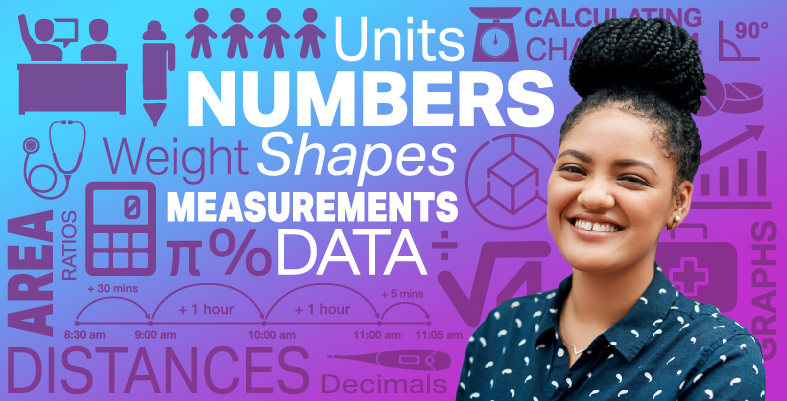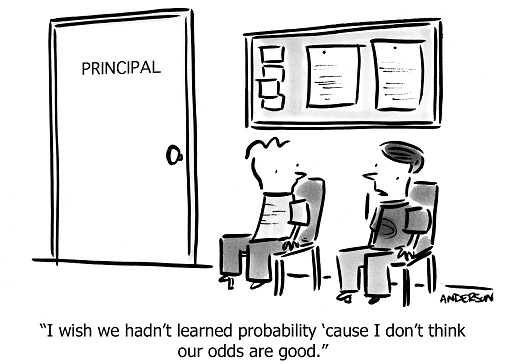Everyday maths for Health and Social Care and Education Support 2

Start this free course now. Just create an account and sign in. Enrol and complete the course for a free statement of participation or digital badge if available.

Free course

# 7 Probability

You will use probability regularly in your day-to-day life:

• Should you take an umbrella out with you today?

• What are the chances of the bus being on time?Figure 23 Probability – the odds are you are already using it

Probability is all about how likely, or unlikely, something is to happen. When you flip a coin for example, the chances of it landing on heads is ½ or 50% or 0.5 – remember from your work in Session 1 how fractions, decimals and percentages can be converted into one another?

The probability, or likelihood, of an event happening is perhaps most easily expressed as a fraction to begin with. Then, if you want to express it as a percentage or a decimal you can just convert it.

Let’s look at an example.

## Example: Chocolate probability

A box of chocolates contains 15 milk chocolates, 5 dark chocolates and 10 white chocolates. If the box is full and you choose a chocolate at random, what is the likelihood of choosing a dark chocolate?

### Method

There are 5 dark chocolates in the box. There are 15 + 5 + 10 = 30 chocolates in the box altogether.

The probability of choosing a dark chocolate is therefore:

=

You could also be asked the probability of choosing either a dark or a white chocolate. For this you just need the total of dark and white chocolates:

5 + 10 = 15

The total number of chocolates in the box remains the same so the likelihood of choosing a dark or a white chocolate is:

=

You could even be asked what the likelihood is of an event not happening. For example, the likelihood that you will not choose a white chocolate. In this case, the total number of chocolates that are not white is 15 + 5 = 20.

Again, the total number of chocolates in the box remains the same and so the probability of not choosing a white chocolate is:

=

Now have a go yourself by completing the short activity below.

## Activity 13: Calculating probability

1. You buy a packet of multi-coloured balloons for a children’s party. You find that there are 26 red balloons, 34 green balloons, 32 yellow balloons and 28 blue balloons.

You take a balloon out of the packet without looking. What is the likelihood of choosing a green balloon?

2. The school you work at has, 350 children. 80 of these children receive free school meals

1. There are 34 green balloons. The total number of balloons is 26 + 34 + 32 + 28 = 120.

The likelihood of choosing a green balloon is therefore:

• = in its simplest form.

2. If there are 80 children who receive free school meals, there are 350 − 80 = 270 children that do not receive free school meals.

As a fraction this is:

To convert to a percentage, you do 270 ÷ 350 × 100 = 77.14% rounded to two d.p.

You’ve now completed Session 3 of your course, congratulations! Before you begin the fourth and final session, try the end-of-session quiz to check your skills.

Remember that when you are checking your answers, you may have gone about the question in a different way. In a real exam it is always important to show your working as even if you don’t arrive at the correct answer you can still gain marks.

## Summary

In this section you have learned:

• that the probability of an event is how likely or unlikely that event is to happen and that this can be expressed as a fraction, decimal or percentage.

FSM_SSH_2